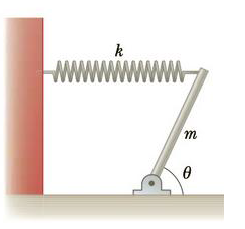Chapter 8, Problem 29P

Chapter
Section
Textbook Problem

Figure P8.29 shows a uniform beam of mass m pivoted at its lower end, with a horizontal spring attached between its top end and a vertical wall. The beam makes an angle θ with the horizontal. Find expressions for (a) the distance d the spring is stretched from equilibrium and (b) the components of the force exerted by the pivot on the beam.Figure P8.29

(a)

To determine
The distance d of the spring stretched from equilibrium.

Explanation

Given Info: The mass of the beam is m, horizontal angle of the beam is θ , and distance of the spring is d.

The following figure shows the free body diagram of the uniform beam,

Formula to calculate the spring force by using Hooke’s law is,

Fs=kd (1)

• k is the spring constant.
• d is the distance of the spring.

The net torque is zero at the lower end of the beam. Therefore,

τ=0Fslsinθmg(l2cosθ)=0

• g is the acceleration due to gravity.
• Fs is the spring force.
• l is the length of the beam.
• θ is the horizontal angle.
• m is the mass of the beam

(b)

To determine
The components of force exerted by the pivot on the beam.

Still sussing out bartleby?

Check out a sample textbook solution.

See a sample solution

The Solution to Your Study Problems

Bartleby provides explanations to thousands of textbook problems written by our experts, many with advanced degrees!

Get Started

Find more solutions based on key concepts